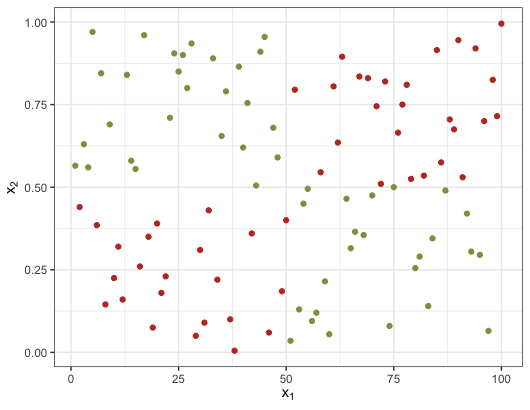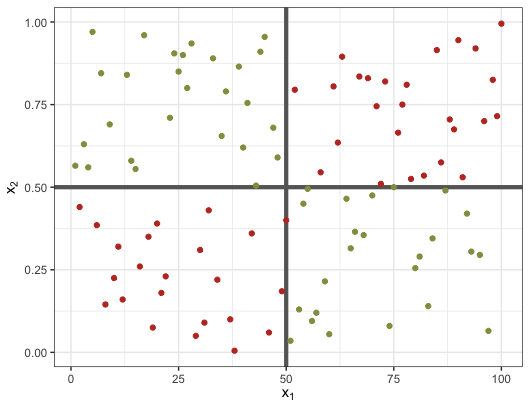# Random Forests by HandBruna Wundervald
brunadaviesw at gmail.com
PhD Candidate in Statistics, Hamilton Institute

# Introduction

Statistical machine learning is a field focused on predictions tasks. When the variable of interest already exists, we are dealing with a supervised problem. The general idea is that we can predict the response variable $$Y$$ based on the information brought by a set of covariables $$\mathbf{X}$$. This is done by fitting a model that relates these predictors to the variable of interest, that can either be quantitative (continuous or discrete) or be a factor variable, with two or more classes.

When the response is discrete, the prediction method is configured as classification method. Classical statistical learning methods for classification are: logistic regression, support vector machines, neural networks, decision trees and random forests (Hastie, Trevor, Tibshirani, Robert, Friedman (2009)). Classification problems emerge in the most different range of occasions, examples include: predict whether someone would pay a bank loan or not based on their financial situation, predict in which party a person someone is more likely to vote based on their political views, ect.

As for the problems involving a quantitative response variable, we call them regression problems. The response can represent infinite different measures, such as human height, human weight, the count of people in a building each day, how long it takes for a bus to arrive at a bus stop, salaries for technology professions, and so on. Some methods used for classification can also be applied in this cases, as neural networks, regression trees and random forests. Other methods for regression include least squares linear regression, survival regression, time series models and several more (Hastie, Trevor, Tibshirani, Robert, Friedman (2009)).

# Regression Trees

Decision trees are flexible non-parametric methods that be can applied to both regression and classification problems. It is based on the estimation of a series of binary conditional statements (if-else) on the predictors space. As an illustration, let us get back to a classification problem demonstrated in Figure 1. On the left we see the plot of two predictor variables $$x_1$$ and $$x_2$$ and the color represents the class to each data point belongs. Suppose the two classes are the name of the colors, “red” and “green”. An optimal way of correctly classyfing the points is shown on the right plot. The rule is: if $$x_1 <= 50$$ and $$x_2 <= 0.5$$ or $$x_1 > 50$$ and $$x_2 > 0.5$$, the point should be classified as “red”; if the point is not in any of these two regions, it should be classified as “green”.Figure 1: Illustration of a decision space

Figure 1 also demonstrates an important characteristic of the trees: its ability to handle non-linear relationships between the predictor variables and the response.

Trees can also be shown as a graph. In Figure 2 we have a simple graph of the rules of our illustration. In this case, the observations in terminal nodes 7 and 4 would be the “red” class, and the nodes 5 and 6 would be the “green” ones.